## Sunday, September 2, 2018

### Random Categorical Imputation

This post is an extension of my previous posts on random imputation: Random Imputation Random vs MICE Immputation
``````library(tidyverse)
set.seed(125)``````
First, simulate some categorical data(a vector of 0’s and 1’s), with a proportion of 68% (or any other proportion) 1’s. Then save the original intact data to be compared later with the same data, but with missing values. The saved data is in effect the counterfactuals, the actual data that we never really know when presented with a dataset containing missing values.
``````cats <- rbinom(10000,1,.68)
cats_all <- cats #save the original intact data - the counterfactuals``````
Now, replace 6000 data with missing values (NA’s). The actual number of NA’s will be less than the requested amount because there will be some duplicate values produced by the random generator.
``````missings <- round(runif(2000, 1, 10000)) #the indices of the missing values
cats[missings] <- NA #replace the known data with missing values
sum(is.na(cats)) #total number of NA's``````
``##  1825``
Subset the data into 0’s and 1’s with a table
``(tab1 <- xtabs(~cats))``
``````## cats
##    0    1
## 2592 5583``````
``tab1[]``
``##  2592``
``tab1[]``
``##  5583``
Calculate the proportion of 1’s to 0’s
``````#the proportion of 1's to 0's in the data that we know
prob <- tab1[]/(tab1[] + tab1[])
paste0("The proportion of 1's to 0's is: ", round(prob, 2))``````
``##  "The proportion of 1's to 0's is: 0.68"``
Replace the missing values using random imputation with binomial distribution.
``````#impute the missing values
cats <- if_else(is.na(cats), rbinom(1,1,prob), cats)``````
Compare each value in the imputed data to its counterpart in the original intact data.
``````toll <- as.numeric(0) #initialize a vector
#compare the conterfactual to the imputed data, 0 = a miss
for(i in seq_along(cats_all)) {
toll[[i]] <- if_else(cats[[i]] == cats_all[[i]], 1, 0)
}``````
How accurate was the imputation compared to the counterfactuals?
``````# number of misses after imputation
paste(sum(toll == 0), "misses")``````
``##  "593 misses"``
``````#accuracy of the imputation
acc  <- 1 - (sum(toll == 0)/length(cats_all))
paste0("The accuracy of the categorical imputation was: ", acc)``````
``##  "The accuracy of the categorical imputation was: 0.9407"``
Translate the above into a function:
``````accuracy <- function(n, prop, missing) {
set.seed(125)
cats <- rbinom(n,1L,prop)
cats_all <- cats
missings <- round(runif(missing, 1, n))
cats[missings] <- NA
tab1 <- xtabs(~cats)
prob <- tab1[]/(tab1[] + tab1[])
cats <- if_else(is.na(cats), rbinom(1,1,prob), cats)
toll <- as.numeric(0)
for(i in seq_along(cats_all)) {
toll[[i]] <- if_else(cats[[i]] == cats_all[[i]], 1, 0)
}
acc  <- 1 - (sum(toll == 0)/length(cats_all))
return(acc)
}``````
Also, create a function to plot the accuracy with different proportions of 0’s and 1’s, and with different amounts of missing values.
``````plotacc <- function(prop) {
for(i in 1:100) {
j = i * 100
acc[i]<- accuracy(10000, prop, j)
append(acc, acc[i])
}
miss <- seq(100, 10000, 100)
acc_df <- data_frame(acc, miss)
acc_df\$index <- seq(1, nrow(acc_df), 1)

title_prop <- paste("prop = ", prop)
ggplot(acc_df) +
geom_line(aes(x =miss, y = acc), color = "blue") +
ggtitle(title_prop) +
ylab("Imputation Accuracy") +
xlab("Number of Missing Data")
}``````
Now let’s plot 10000 data varying the proportion of 1’s and the total number of missing data. We’ll start right in the middle at prop = 0.50.
``plotacc(0.50)``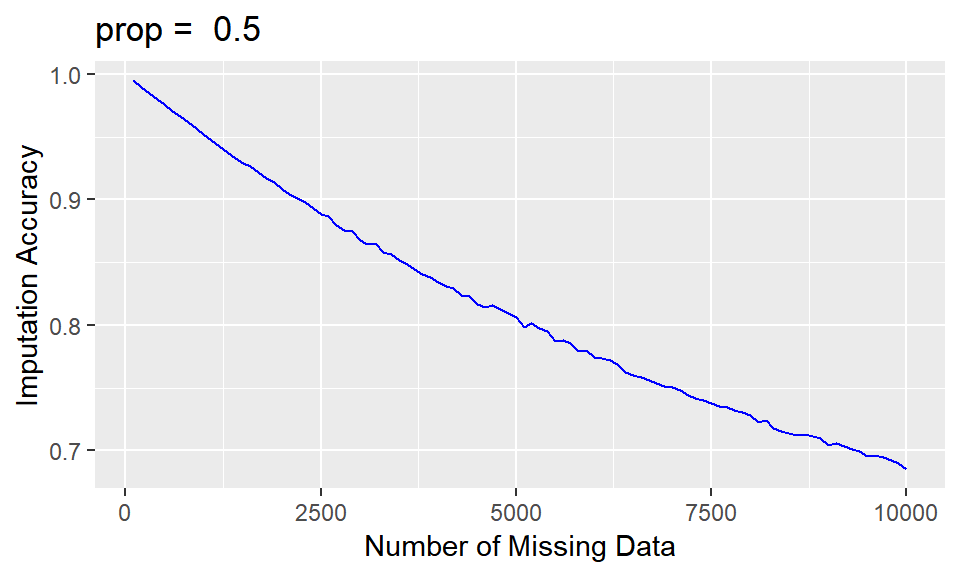This method has a peculiar property in that as we vary the proportion of 0’s and 1’s away from 0.50, there appear non-periodic negative spikes which increase in amplitude and decrease in frequency as we approach 1.00 and 0.00. The trend also becomes more horizontal with progression away from 0.50.
``plotacc(.55)``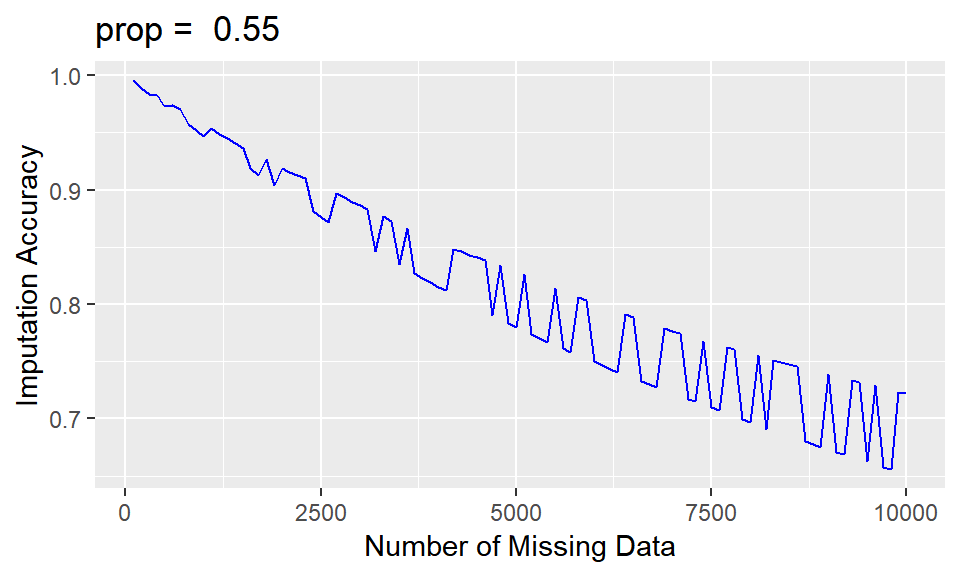``plotacc(.60)``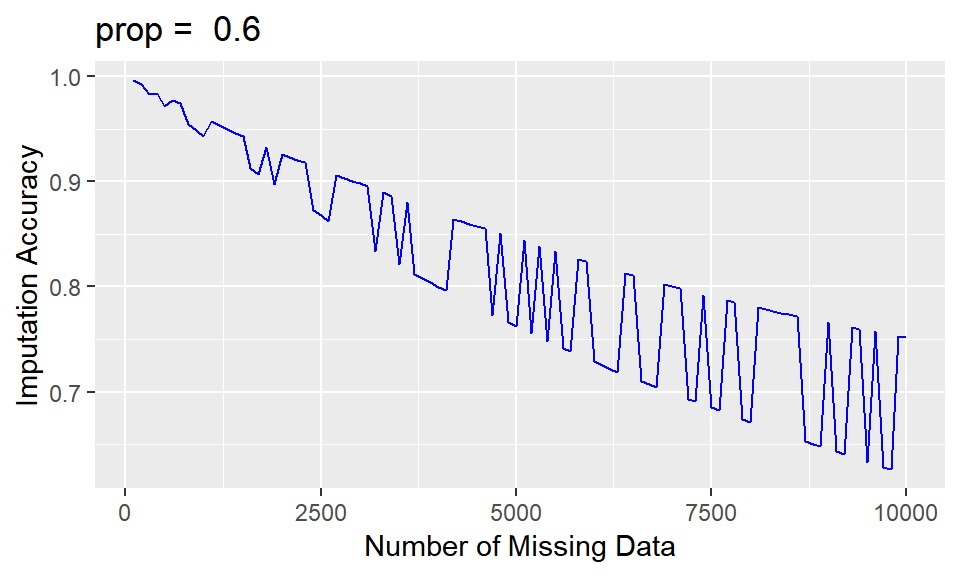``plotacc(.70)``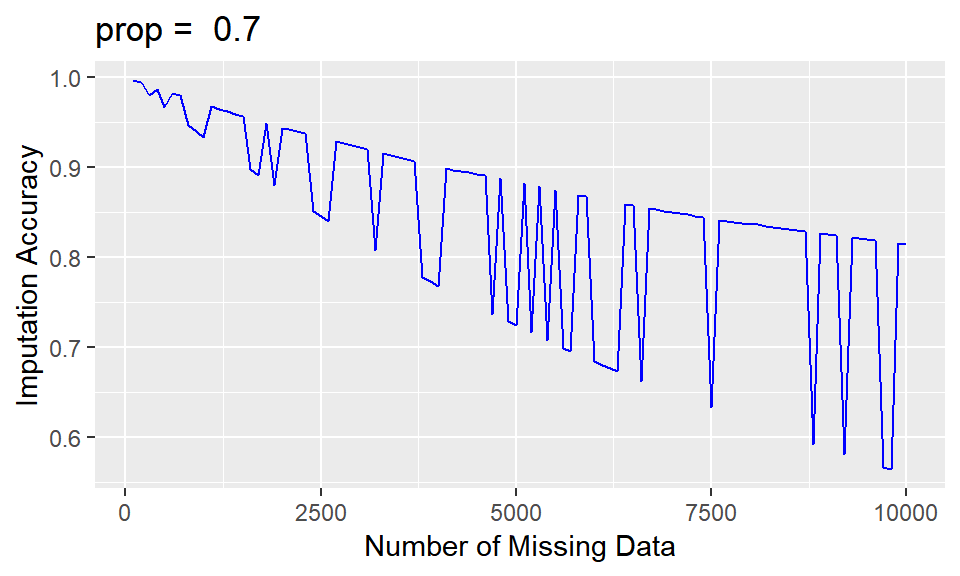``plotacc(.80)``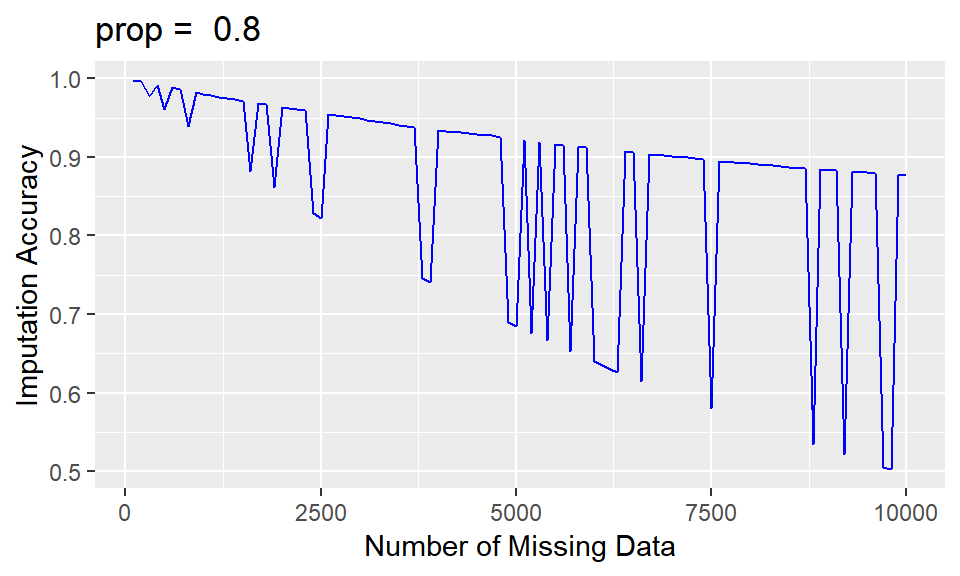``plotacc(.90)``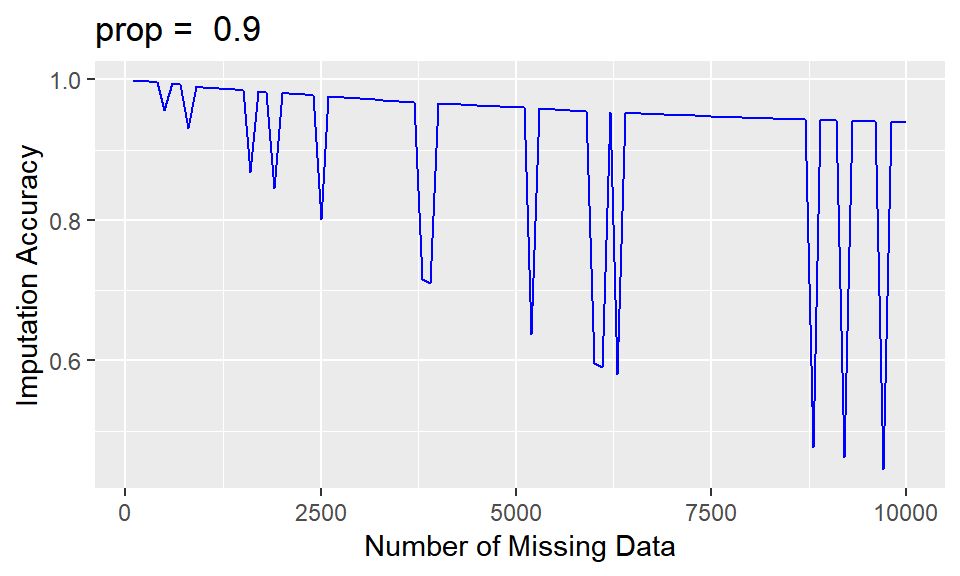``plotacc(.99)``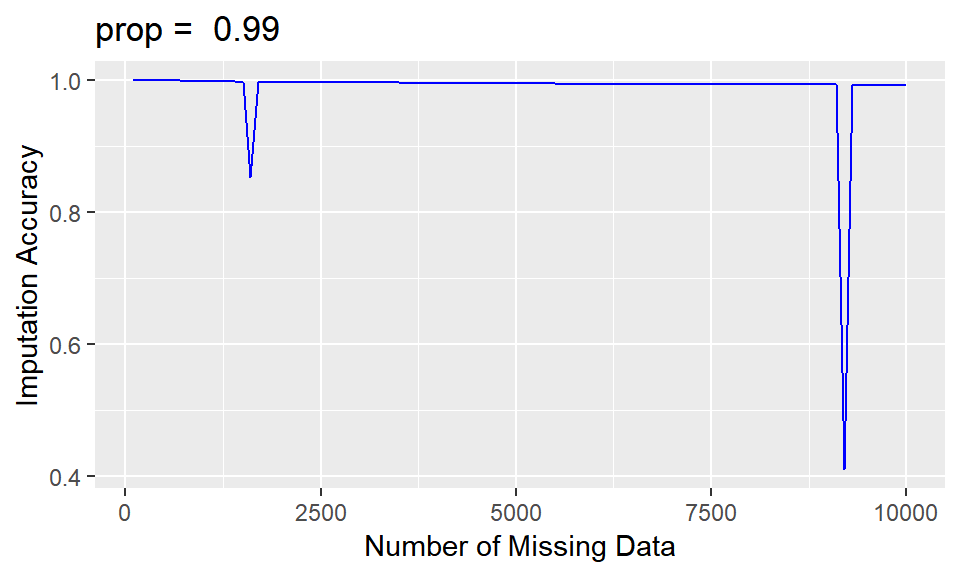Now going in the opposite direction:
``plotacc(.45)``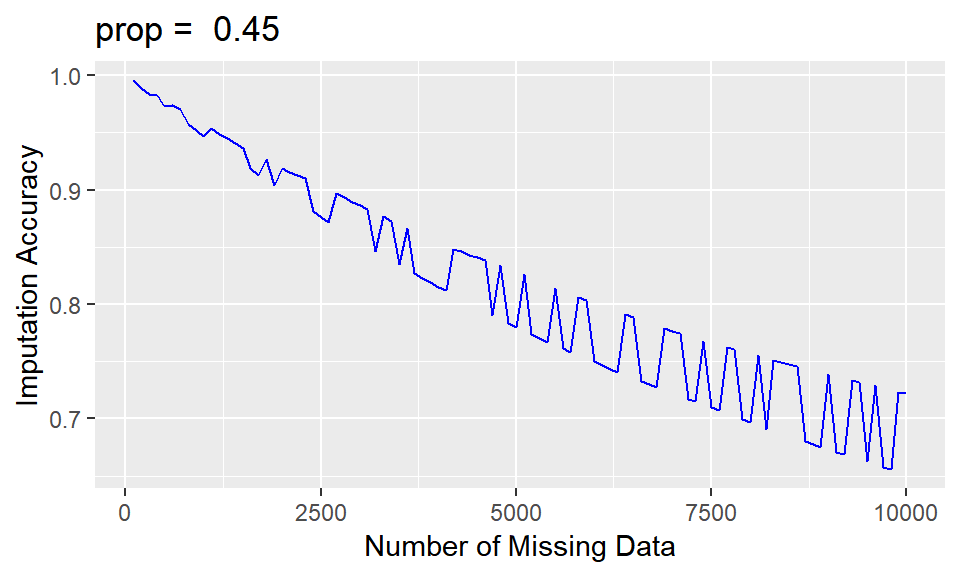``plotacc(.40)``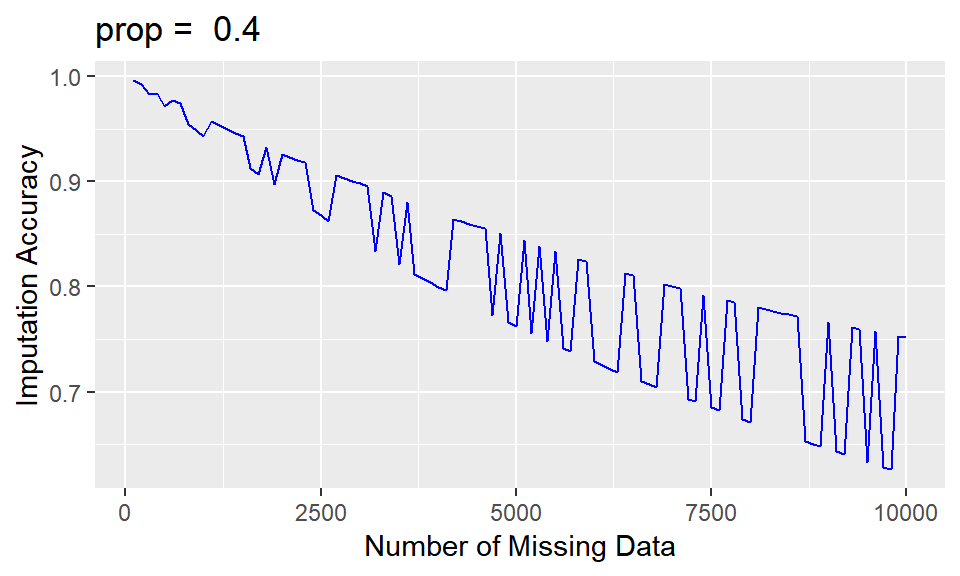``plotacc(.30)``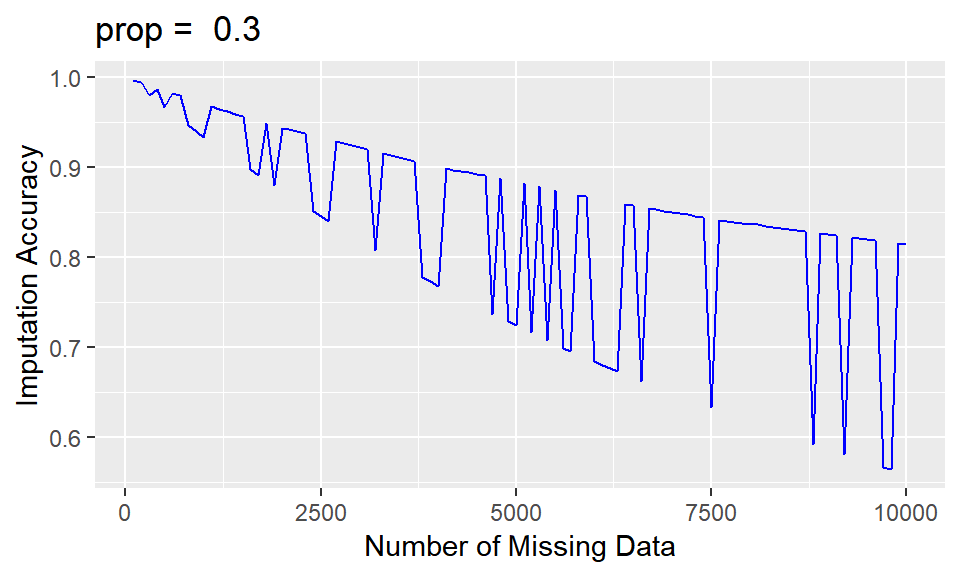``plotacc(.20)``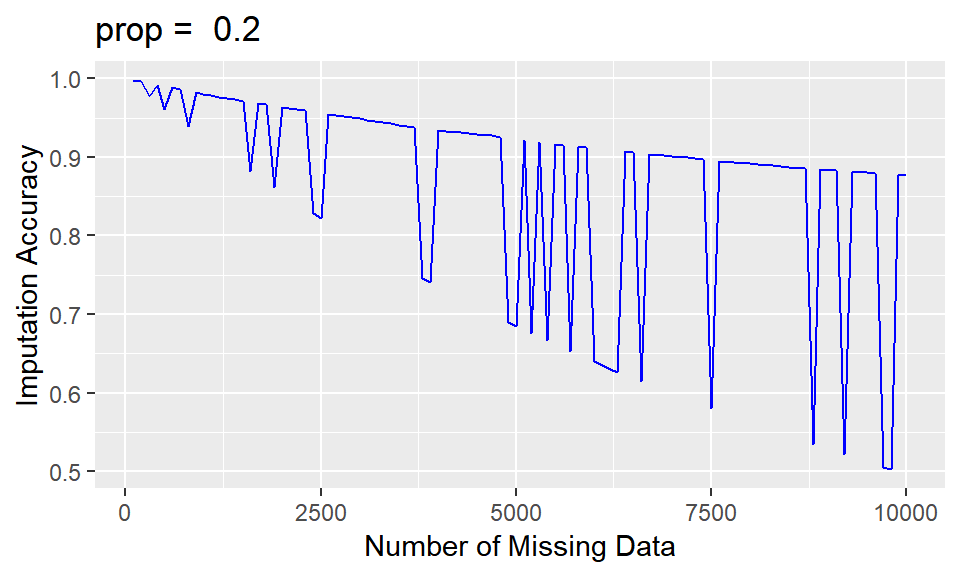``plotacc(.10)``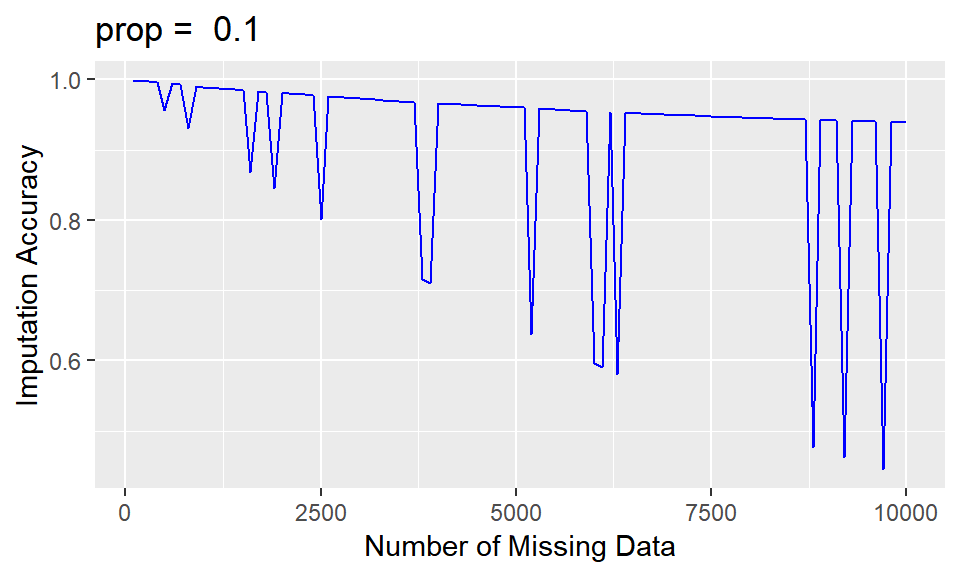``plotacc(.01)``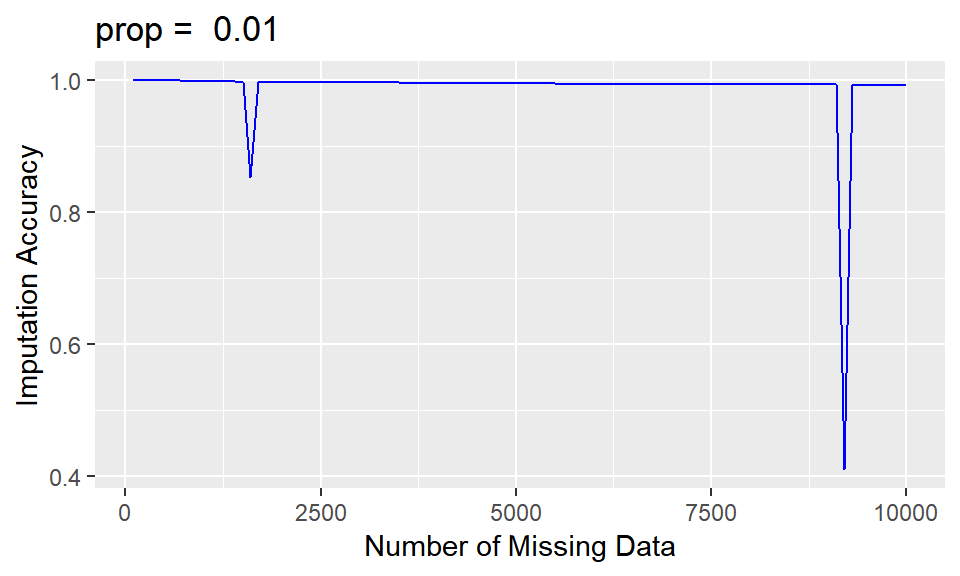I don’t have an explanation for the spikes. Their frequency seems to be random. Perhaps someone would like to comment on their origin.
This method appears to be a highly accurate method for imputing categorical missing data, especially when we are dealing with 30% or less missing data. Granted that a fair coin toss should give around 50% accuracy over the long term, but random imputation may be acceptable for imputation in many situations.
``sessionInfo()``
``````## R version 3.5.1 (2018-07-02)
## Platform: x86_64-w64-mingw32/x64 (64-bit)
## Running under: Windows 10 x64 (build 17134)
##
## Matrix products: default
##
## locale:
##  LC_COLLATE=English_United States.1252
##  LC_CTYPE=English_United States.1252
##  LC_MONETARY=English_United States.1252
##  LC_NUMERIC=C
##  LC_TIME=English_United States.1252
##
## attached base packages:
##  stats     graphics  grDevices utils     datasets  methods   base
##
## other attached packages:
##  forcats_0.3.0   stringr_1.3.1   dplyr_0.7.6     purrr_0.2.5
##  readr_1.1.1     tidyr_0.8.1     tibble_1.4.2    ggplot2_3.0.0
##  tidyverse_1.2.1
##
## loaded via a namespace (and not attached):
##   Rcpp_0.12.18     cellranger_1.1.0 pillar_1.3.0     compiler_3.5.1
##   plyr_1.8.4       bindr_0.1.1      tools_3.5.1      digest_0.6.16
##   lubridate_1.7.4  jsonlite_1.5     evaluate_0.11    nlme_3.1-137
##  gtable_0.2.0     lattice_0.20-35  pkgconfig_2.0.2  rlang_0.2.2
##  cli_1.0.0        rstudioapi_0.7   yaml_2.2.0       haven_1.1.2
##  bindrcpp_0.2.2   withr_2.1.2      xml2_1.2.0       httr_1.3.1
##  knitr_1.20       hms_0.4.2        rprojroot_1.3-2  grid_3.5.1
##  tidyselect_0.2.4 glue_1.3.0       R6_2.2.2         readxl_1.1.0
##  rmarkdown_1.10   modelr_0.1.2     magrittr_1.5     backports_1.1.2
##  scales_1.0.0     htmltools_0.3.6  rvest_0.3.2      assertthat_0.2.0
##  colorspace_1.3-2 labeling_0.3     stringi_1.1.7    lazyeval_0.2.1
##  munsell_0.5.0    broom_0.5.0      crayon_1.3.4``````